# serhii.net

In the middle of the desert you can say anything you want

• ### Day 1606 (25 May 2023)

#### Pandas seaborn pretty correlation code

Old code I wrote for making ds.corr() more readable, looked for it three times already ergo its place is here.

Basically: removes all small correlations, and optionally plots a colorful heatmap of that.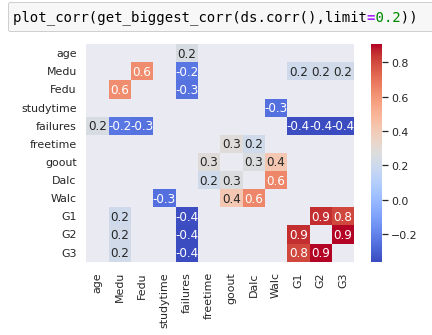def plot_corr(res:pd.DataFrame):
import seaborn as sns
sns.heatmap(res, annot=True,fmt=".1f",cmap="coolwarm")

def get_biggest_corr(ds_corr: pd.DataFrame, limit: float=0.8, remove_diagonal=True, remove_nans=True,plot=False) -> pd.DataFrame:
res =  ds_corr[(ds_corr>limit) | (ds_corr<-limit)]
if remove_diagonal:
np.fill_diagonal(res.values, np.nan)
if remove_nans:
res = res.dropna(how='all', axis=0)
res = res.dropna(how='all', axis=1)
if plot:
plot_corr(res)
else:
return res


## Intro

I like seaborn but kept googling the same things and could never get any internal ‘consistency’ in it, which led to a lot of small unsystematic posts1 but I felt I was going in circles. This post is an attempt to actually read the documentation and understand the underlying logic of it all.

I’ll be using the context of my “Informationsvisualisierung und Visual Analytics 2023” HSA course’s “Aufgabe 6: Visuelle Exploration multivariater Daten”, and the dataset given for that task: UCI Machine Learning Repository: Student Performance Data Set:

This data approach student achievement in secondary education of two Portuguese schools. The data attributes include student grades, demographic, social and school related features) and it was collected by using school reports and questionnaires

Goal:

• Mental picture of the different important architectural parts (figure/axis-level functions)
• Clarity about where are matplotlib things exposed
• Central place for the things I need every time I do seaborn stuff, that are currently distributed in many small posts

I’m not touching the seaborn.objects interface as the only place I’ve seen it mentioned is the official docu and I’m not sure it’s worth digging into for now.

## Basics

An introduction to seaborn — seaborn 0.12.2 documentation

### Themes and setting the (default) theme

# sets default theme that looks nice
# and used in all pics of the tutorial
sns.set_theme()


## Figure-level vs. axes-level functions2

Overview of seaborn plotting functions — seaborn 0.12.2 documentation: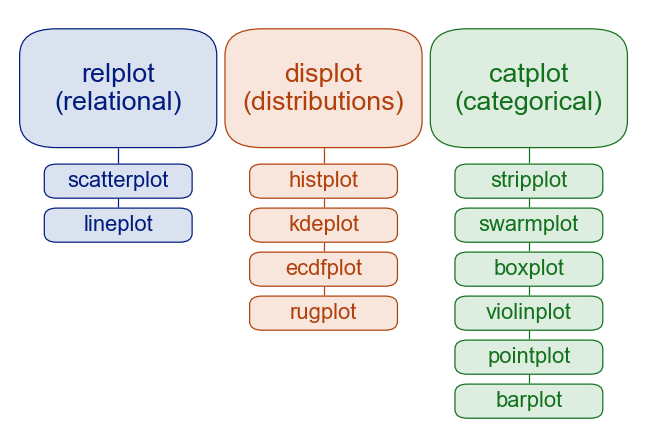### Basics

Functions can be:

• “axes-level”: They plot data onto a single matplotlib.axes.Axes object and return it
• Contains the legend on the plot
• The axes-level functions are written to act like drop-in replacements for matplotlib functions. While they add axis labels and legends automatically, they don’t modify anything beyond the axes that they are drawn into. That means they can be composed into arbitrarily-complex matplotlib figures with predictable results.

• “figure-level”: interface through a seaborn object that manages the figure
• (usually a FacetGrid)
• Each module has a single figure-level function that creates/accesses axes-level ones (through the kind=xxx parameter)
• Have the col= and row= params that automatically create subplots!
• They take care of their own legend
• The figure-level functions wrap their axes-level counterparts and pass the kind-specific keyword arguments (such as the bin size for a histogram) down to the underlying function. That means they are no less flexible, but there is a downside: the kind-specific parameters don’t appear in the function signature or docstring

Special cases:

• sns.jointplot()3 has one plot with distributions around it and is a JointGrid
• sns.pairplot()4 “visualizes every pairwise combination of variables simultaneously” and is a PairGrid

In the pic above, the figure-level functions are the blocks on top, their axes-level functions - below. (TODO: my version of that pic with the kind=xxx bits added)

### Customization

#### Figure-level

The returned seaborn.FacetGrid can be customized in some ways (all examples here from that documentation link).

##### FacetGrid customization params
g.map_dataframe(sns.scatterplot, x="total_bill", y="tip")
g.set_axis_labels("Total bill ($)", "Tip ($)")
g.set_titles(col_template="{col_name} patrons", row_template="{row_name}")
g.set(xlim=(0, 60), ylim=(0, 12), xticks=[10, 30, 50], yticks=[2, 6, 10])
g.tight_layout()
g.savefig("facet_plot.png")

##### Accessing underlying matplotlib objects

It’s possible to access the underlying matplotlib axes:

g = sns.FacetGrid(tips, col="sex", row="time", margin_titles=True, despine=False)
g.map_dataframe(sns.scatterplot, x="total_bill", y="tip")
for (row_val, col_val), ax in g.axes_dict.items():
if row_val == "Lunch" and col_val == "Female":
ax.set_facecolor(".95")
else:
ax.set_facecolor((0, 0, 0, 0))


And generally access matplotlib stuff:

• ax: The matplotlib.axes.Axes when no faceting variables are assigned.
• axes: An array of the matplotlib.axes.Axes objects in the grid.
• axes_dict: A mapping of facet names to corresponding matplotlib.axes.Axes.
• figure: Access the matplotlib.figure.Figure object underlying the grid (formerly fig)
• legend: The matplotlib.legend.Legend object, if present.
##### FacetGrid.set()

(Previously: 230515-2257 seaborn setting titles etc. with matplotlib set)

FacetGrid.set() is used from time to time in the tutorial (e.g. .set(title="My title"), especially in Building structured multi-plot grids) but never explicitly explained; in its documentation, there’s only “Set attributes on each subplot Axes”.

It sets attributes for each subplot’s matplotlib.axes.Axes. Useful ones are:

• title for plot title (set_title())
• xticks,yticks
• set_xlabel(), set_ylabel (but not sequentially as return value is not the ax)

#### Axis-level functions + adding them to a matplotlib Figure

Axis-level functions “can be composed into arbitrarily complex matplotlib figures”.

Practically:

fig, axs = plt.subplots(2)
sns.heatmap(..., ax=axs)
sns.heatmap(..., ax=axs)


### Specifying figure sizes

Documentation has an entire section on it5, mostly reprasing and stealing screenshots from it.

#### Axis-level

For axis-level functions, the size of the plot is determined by the size of the Figure it is part of and the axes layout in that figure. You basically use what you would do in matplotlib, relevant being:

### Figure-level functions

TL;DR they have FacetGrid’s' height= and aspect=(ratio; 0.75 means 5 cells high, 4 cells wide) params that work per subplot.

Figure-level functions' size has differences:

• the functions themselves have parameters to control the figure size (although these are actually parameters of the underlying FacetGrid that manages the figure)
• these parameters, height and aspect, work like this: width = height * aspect
• by default, subplots are square
• The parameters correspond to the size of each subplot, not the overall figure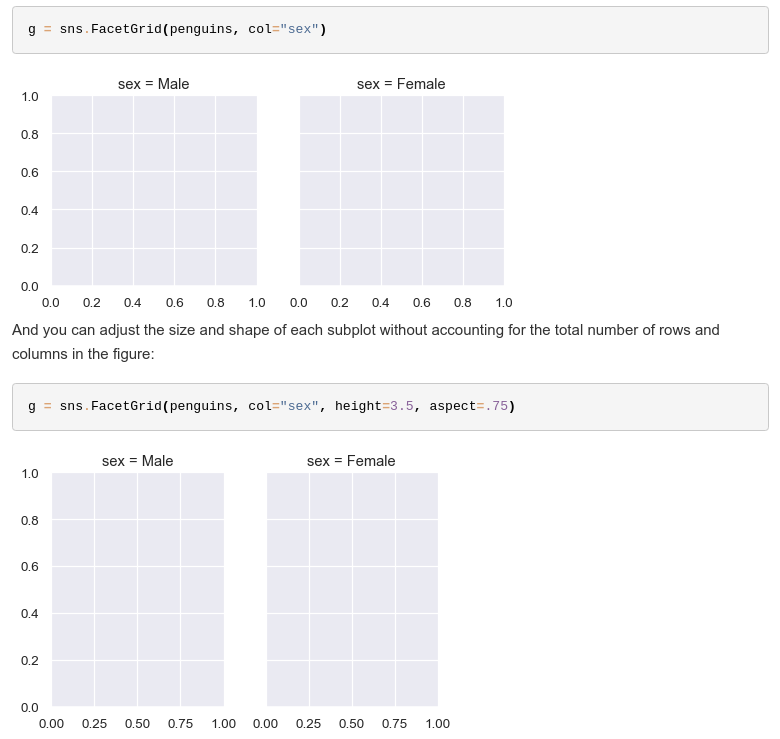## Modules

Blocks doing similar kinds of plots, each with a figure-level function and multiple axis-level ones. Listed in the API reference.6

• Distribution plots
• displot is the figure-level interface
• ! $\neq$ disTplot that is deprecated
•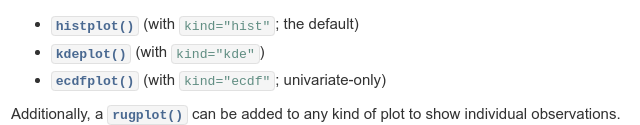• histplot: Plot univariate or bivariate histograms to show distributions of datasets.
• kdeplot :Plot univariate or bivariate distributions using kernel density estimation.
• Less useful to me now:
• ecdfplot: Plot empirical cumulative distribution functions.
• rugplot: add ticks to axes with the distribution, usually in addition to other plots
• Categorical plots
• seaborn.catplot is the figure-level interface
•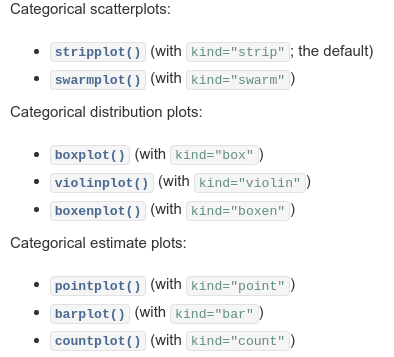• Regression plots
• seaborn.relplot
• scatterplot (with kind="scatter"; the default)
• lineplot (with kind="line")
• Matrix plots

And again, the already mentioned special cases, now with pictures:

• sns.jointplot()3 has one plot with distributions around it and is a JointGrid: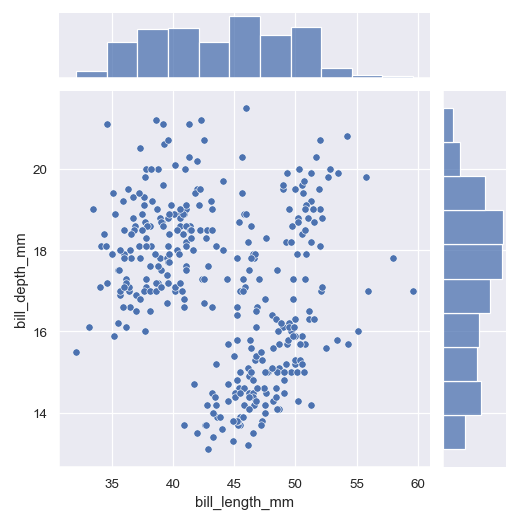• sns.pairplot()4 “visualizes every pairwise combination of variables simultaneously” and is a PairGrid: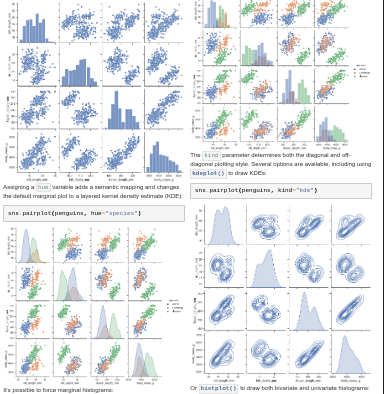## Design

### Marks

The parameters for marks are described better in the tutorial than I ever could: Properties of Mark objects — seaborn 0.12.2 documentation:

• Coordinates
• Colors
• Marker/line styles
• Size
• Text
• Align, size, offset

TODO my main remaining question is where/how do I set this? Can this be done outside the seaborn.objects interface I don’t want to learn.

### Markers

• Marker size Pass e.g. s=30 to the plotting function. (size= would be a column name)
• Marker style: you are infinitely flexible actually! And this even goes in the legend
•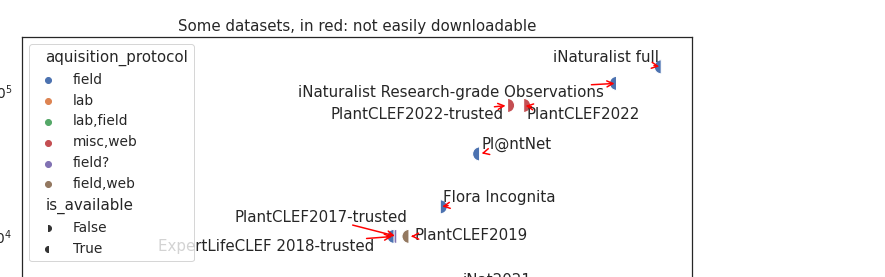sns.scatterplot(

style="is_available",
# marker=MarkerStyle("o", "left"),
markers={True: MarkerStyle("o", "left"), False: MarkerStyle("o", "right")},
)


## Individual questions/topics

### Colors, palettes, themes etc

#### Setting theme and context

Controlling figure aesthetics — seaborn 0.12.2 documentation

There are five preset seaborn themes: dark, white, ticks, whitegrid, darkgrid. This picture contains the first four of the above in this order.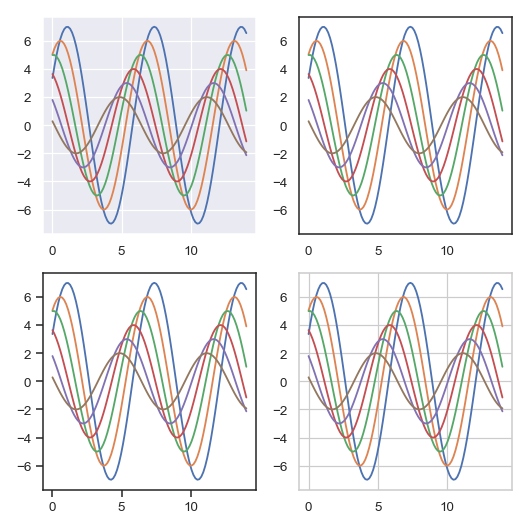set_context()

#### Color palettes

The tutorial has this: Choosing color palettes — seaborn 0.12.2 documentation with both a theoretical basis about color and stuff, and the “how to set it in your plot”.

TL;DR sns.color_palette(PALETTE_NAME, NUM_COLORS, as_cmap=TRUE_IF_CONTINUOUS)

seaborn.color_palette() returns a list of colors or a continuous matplotlib ListedColormap colormap:

• Accepts as palette, among other things:

• Name of a seaborn palette (deep, muted, bright, pastel, dark, colorblind)
• Name of matplotlib colormap
• ‘light:<color>’, ‘dark:<color>’, ‘blend:<color>,<color>’
• A sequence of colors in any format matplotlib accepts
• n_colors: will truncate if it’s less than palette colors, will extend/cycle palette if it’s more

• as_cmap - whether to return a continuous ListedColormap

• desat

• You can do .as_hex() to get the list as hex colors.

• You can use it as context manager: with sns.color_palette(...): to temporarily change the current defaults.

#### Reversing palettes/colormaps

Matplotlib colormap + _r (tab10_r).

I needed a colormap where male is blue and female is orange, tab10 has these colors but in reversed order. This is how I got a colormap with the first two colors but reversed:

cm = sns.color_palette("tab10",2)[::-1]


First I generated a color_palette of 2 colors, then reversed the list of tuples it returned.

## Individual plot types

### Distributions

#### Plotting multiple distributions on the same subplot

• histplot has different approaches for plotting multiple= distributions on the same plot:
• layer (default, make them overlap)
• stack (one on top of the other)
• dodge (multiple small columns for each distribution):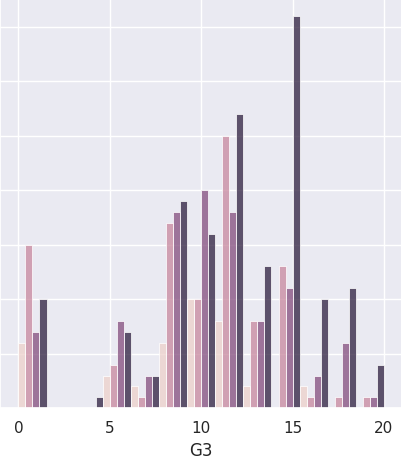• fill (this beauty):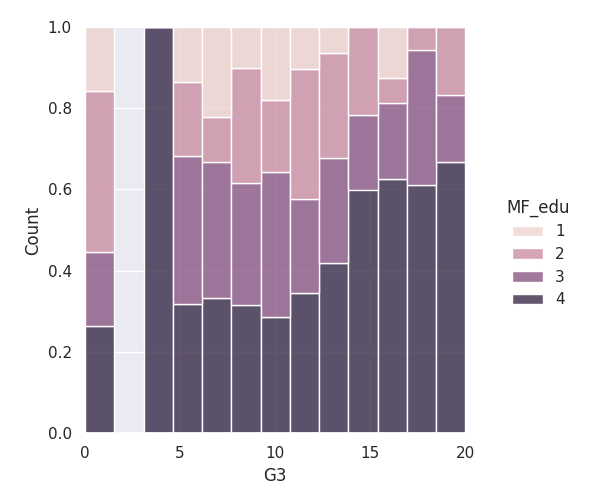• KDEplot can do this too! multiple=fill

### Categorical

#### Pointplot

seaborn.pointplot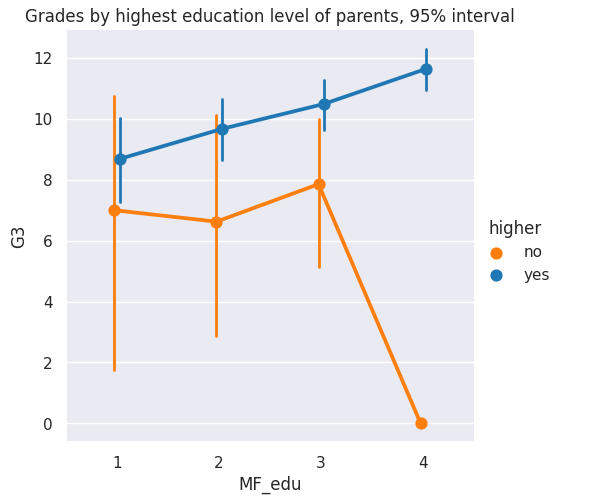• Errorbars:
• To make the errorbars not-overlap, dodge=True
• You can control their width through errwidth=
• Statistical estimation and error bars — seaborn 0.12.2 documentation has a really cool and thorough description of the types and theory:

The error bars around an estimate of central tendency can show one of two general things: either the range of uncertainty about the estimate or the spread of the underlying data around it. These measures are related: given the same sample size, estimates will be more uncertain when data has a broader spread. But uncertainty will decrease as sample sizes grow, whereas spread will not.

### Random

#### Heatmaps

• To order the rows/columns, you have to use pandas’s pd.sort_index()
• To annotate / add text to the cells: annot=True, fmt=".1f"
• To change the range of the colorbar/colormap , use vmin=/vmax=

Previously: Small unsystematic posts about seaborn: - Architecture-ish: - 230515-2257 seaborn setting titles etc. with matplotlib set - 230515-2016 seaborn things built on FacetGrid for easy multiple plots - Small misc: - 230428-2042 Seaborn basics - 230524-2209 Seaborn visualizing distributions and KDE plots)

• ### Day 1605 (24 May 2023)

#### Seaborn distributions and KDE plots

• TIL KDE means “Kernel Density Estimation”
• common_norm=True by default applies the same normalization to the entire distribution. False scales each independently. This is critical in many cases, esp. with stat="probability"

Generally: I read the seaborn documentation, esp. the high level architecture things, and a lot of things I’ve been asking myself since forever (e.g. 230515-2257 seaborn setting titles etc. with matplotlib set) have become much clearer - and will be its own post. I love seaborn and it’s honestly worth learning to use well and systematically.

• ### Day 1604 (23 May 2023)

#### Huggingface datasets can become pandas dataframes

ds = Dataset(...)
ds.set_format("pandas")


#### More matplotlib color fun with cycler package

There’s cycler, a package:

It returns cycles of dicts, finite or infinite:

from cycler import cycler

# list of colors
pal = sns.color_palette("Paired")

# cycler is a finite cycle, cycler() is an infinite
cols = iter(cycler(color=pal)())

# every time you need a color
my_color = next(cols)


• ### Day 1597 (16 May 2023)

#### matplotlib datetime axes indexing

If you have an axis indexed from timestamps and want to draw stuff there, you need to convert between datetimes and coordinates.

from matplotlib.dates import date2num
coordinate_float_value = date2num(your_timestamp)
# there's also num2date, etc.


Then you can do things like

g=sns.histplot(...)

g.axes.bar(x=date2num(timestamp),height=5,width=0.01)

#or


Ref:

And, for later, gridlnes/dates fun I’ll document later maybe:

from matplotlib.dates import date2num, drange
from datetime import timedelta
import matplotlib.ticker as ticker

g = sns.lineplot(...)

# We create a grid located at midnight of each day
t_end = df_item['time.pull'].max().ceil(freq="D")
t_start = df_item['time.pull'].min().floor(freq="D")
dr_days = drange(t_start,t_end,delta=timedelta(days=1))
dr_hours = drange(t_start,t_end,delta=timedelta(hours=4))

g.axes.grid(True, axis='both',which='major')
g.axes.grid(True, axis='both',which='minor',linewidth=0.2,linestyle="--")
g.axes.xaxis.set_major_locator(ticker.FixedLocator(dr_days))
g.axes.xaxis.set_minor_locator(ticker.FixedLocator(dr_hours))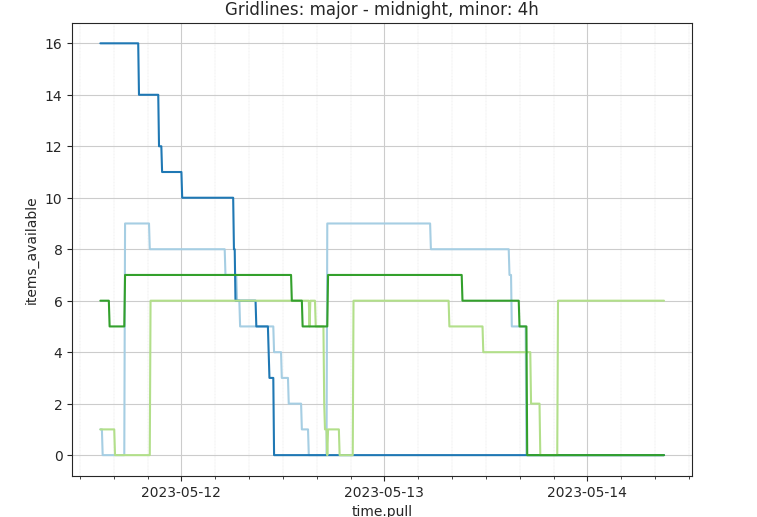• ### Day 1596 (15 May 2023)

#### Seaborn setting titles and stuff through matplotlib's axis .set() function

For titles I was using sns.histplot(..).set(title="My title"), but I couldn’t find any documentation for that .set() function in the seaborn docu.

It’s actually a matplotlib function!

(TODO: understand much better how seaborn exposes matplotlib’s internals. Then I can google for matplotlib stuff too)

### Bonus: setting figure title for each facet of a FacetGrid AND the entire figure

You can access the matplotlib Figure through .fig , then use matplotlib.pyplot.suptitle — Matplotlib 3.7.1 documentation for the main figure title!

x = sns.displot(
data=xxx,
x='items_available',
col="item.item_category",
).set_titles(col_template="{col_name}") # Title template for each facet

# Main figure title, through matplotlib Figure
x.fig.suptitle("Distribution of sums of all items_available per time.pull",va='bottom')


#### seaborn things built on FacetGrid for easy multiple plots

This is REALLY neat and seaborn is now officially the best thing since sliced bread (only having pie charts could make it better1).

relplot Combine a relational plot and a FacetGrid

displot Combine a distribution plot and a FacetGrid

catplot Combine a categorical plot and a FacetGrid

lmplot Combine a regression plot and a FacetGrid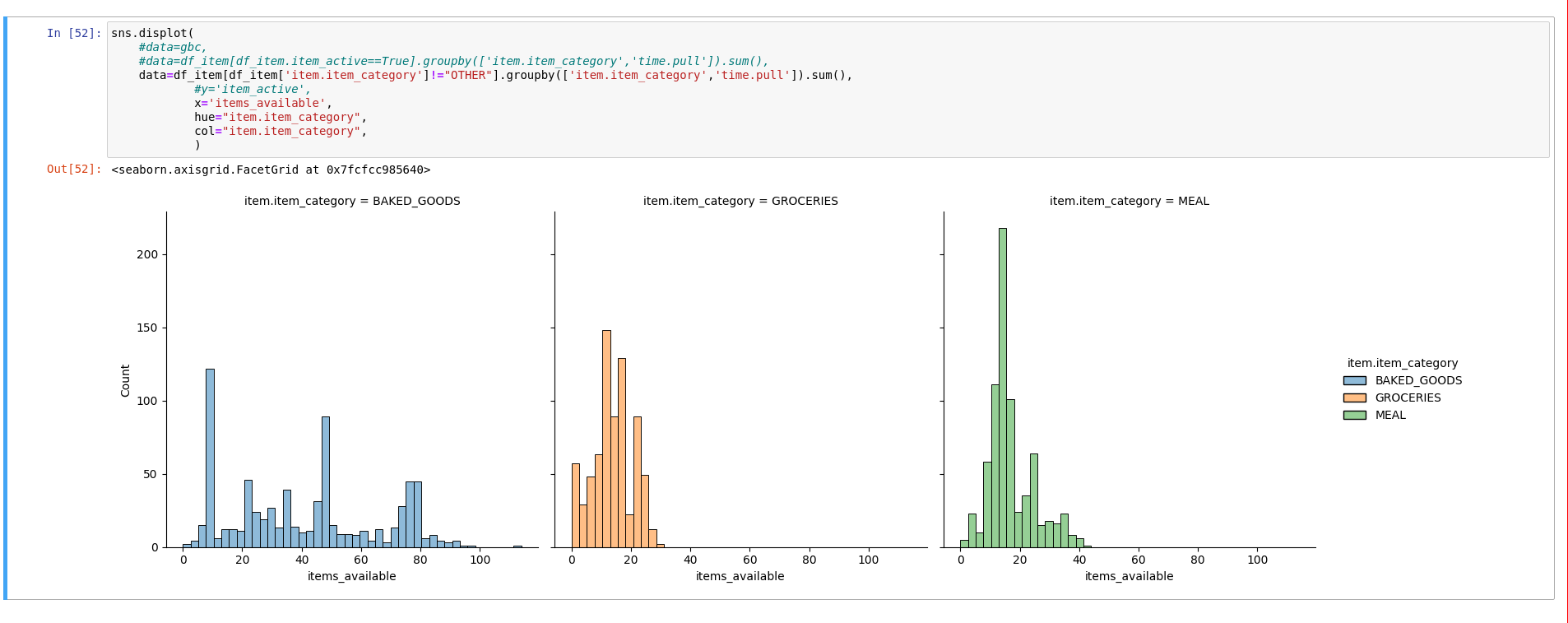sns.displot(
data=df_item[df_item['item.item_category']!="OTHER"].groupby(['item.item_category','time.pull']).sum(),
#y='item_active',
x='items_available',
hue="item.item_category",
col="item.item_category",
)


All of this takes row/col arguments that neatly create separate plots!

1. Obyde/obsidian internal link test: 230515-1855 Pie charts considered harmful ↩︎

#### Pie Charts Considered Harmful

Note that seaborn doesn’t create pie charts, as seaborn’s author considers those to be unfit for statistical visualization. See e.g. Why you shouldn’t use pie charts – Johan 1

Pies and doughnuts fail because:

• Quantity is represented by slices; humans aren’t particularly good at estimating quantity from angles, which is the skill needed.
• Matching the labels and the slices can be hard work.
• Small percentages (which might be important) are tricky to show.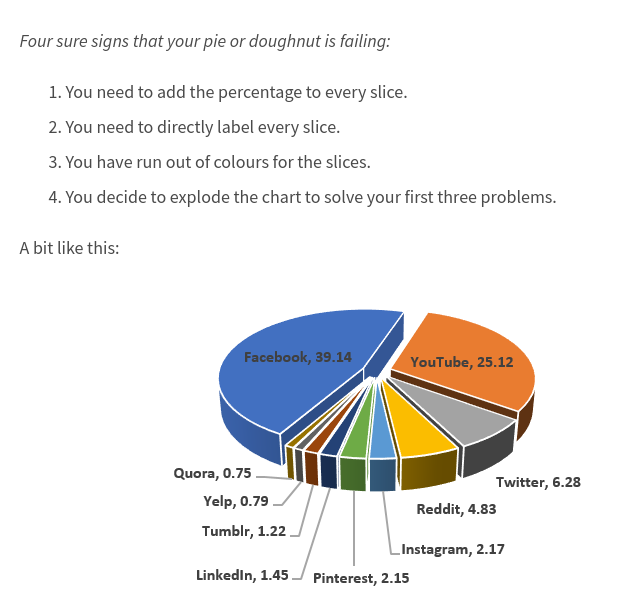The world is interesting.

#### Pandas set column value based on (incl.groupby) filter

TL;DR

df.loc[row_indexer, col_indexer] = value


col_indexer can be a non-existing-yet column! And row_indexer can be anything, including based on a groupby filter.

Below, the groupby filter has dropna=False which would return also the rows that don’t match the filter, giving a Series with the same indexes as the main df

# E.g. this groupby filter - NB.  dropna=False
df_item.groupby(['item.item_id']).filter(lambda x:x.items_available.max()>0, dropna=False)['item.item_id']

# Then we use that in the condition, nice arbitrary example with item.item_id not being the index of the DF
df_item.loc[df_item['item.item_id']==df_item.groupby(['item.item_id']).filter(lambda x:x.items_available.max()>0, dropna=False)['item.item_id'],'item_active'] = True


I’m not sure whether this is the “best” way to incorporate groupby results, but seems to work OK for now.

Esp. the remaining rows have nan instead of False, can be worked around but is ugly:

df_item['item_active'] = df_item['item_active'].notna()

# For plotting purposes
sns.histplot(data=df_item.notna(), ... )


Pandas Filter by Column Value - Spark By {Examples} has more examples of conditions:

# From https://sparkbyexamples.com/pandas/pandas-filter-by-column-value/

df.loc[df['Courses'] == value]
df.loc[df['Courses'] != 'Spark']
df.loc[df['Courses'].isin(values)]
df.loc[~df['Courses'].isin(values)]
df.loc[(df['Discount'] >= 1000) & (df['Discount'] <= 2000)]
df.loc[(df['Discount'] >= 1200) & (df['Fee'] >= 23000 )]

df[df["Courses"] == 'Spark']
df[df['Courses'].str.contains("Spark")]
df[df['Courses'].str.lower().str.contains("spark")]
df[df['Courses'].str.startswith("P")]